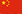Welcome To Our Company中文
You are here: » » What Concentration Means in Chemistry

# What Concentration Means in Chemistry

Views: 13     Author: Anne Marie Helmenstine, Ph.D.     Publish Time: 2020-05-27      Origin: ThoughtCo.

Concentration Definition (Chemistry)

What Concentration Means in Chemistry

By Anne Marie Helmenstine, Ph.D.

Updated February 12, 2020In chemistry, the word "concentration" relates to the components of a mixture or solution. Here is the definition of concentration and a look at different methods used to calculate it.

Concentration Definition

In chemistry, concentration refers to the amount of a substance in a defined space. Another definition is that concentration is the ratio of solute in a solution to either solvent or total solution. Concentration is usually expressed in terms of mass per unit volume. However, the solute concentration may also be expressed in moles or units of volume. Instead of volume, concentration may be per unit mass. While usually applied to chemical solutions, concentration may be calculated for any mixture.

Unit Examples of Concentration: g/cm3, kg/l, M, m, N, kg/L

How to Calculate Concentration

Concentration is determined mathematically by taking the mass, moles, or volume of solute and dividing it by the mass, moles, or volume of solution (or, less commonly, the solvent). Some examples of concentration units and formulas include:

• Molarity (M) - moles of solute/liters of solution (not solvent!)

• Mass Concentration (kg/m3 or g/L) - mass of solute/volume of solution

• Normality (N) - grams active solute/liters of solution

• Molality (m) - moles of solute/mass of solvent (not mass of solution!)

• Mass Percent (%) - mass solute/mass solution x 100% (mass units are the same unit for both solute and solution)

• Volume Concentration (no unit) - volume of solute/volume of mixture (same units of volume for each)

• Number Concentration (1/m3) - number of entities (atoms, molecules, etc.) of a component divided by the total volume of the mixture

• Volume Percent (v/v%) - volume solute/volume solution x 100% (solute and solution volumes are in the same units)

• Mole Fraction (mol/mol) - moles of solute/total moles of species in the mixture

• Mole Ratio (mol/mol) - moles of solute/total moles of all other species in the mixture

• Mass Fraction (kg/kg or parts per) - mass of one fraction (could be multiple solutes)/total mass of the mixture

• Mass Ratio (kg/kg or parts per) - mass of solute/mass of all other constituents in the mixture

• PPM (parts per million) - a 100 ppm solution is 0.01%. The "parts per" notation, while still in use, has largely been replaced by mole fraction

• PPB (parts per billion) - typically used to express contamination of dilute solutions

Some units may be converted from one to another. However, it's not always a good idea to convert between units based on the volume of solution to those based on mass of solution (or vice versa) because volume is affected by temperature.

Strict Definition of Concentration

In the strictest sense, not all means of expressing the composition of a solution or mixture fall under the simple term "concentration". Some sources only consider mass concentration, molar concentration, number concentration, and volume concentration to be true units of concentration.

Concentration Versus Dilution

Two related terms are concentrated and dilute. Concentrated refers to chemical solutions that have high concentrations of a large amount of solute in the solution. If a solution is concentrated to the point where no more solute will dissolve in the solvent, it is said to be saturated. Dilute solutions contain a small amount of solute compared with the amount of solvent.

In order to concentrate a solution, either more solute particles must be added or some solvent must be removed. If the solvent is nonvolatile, a solution may be concentrated by evaporating or boiling off solvent.

Dilutions are made by adding solvent to a more concentrated solution. It's common practice to prepare a relatively concentrated solution, called a stock solution, and use it to prepare more dilute solutions. This practice results in better precision than simply mixing up a dilute solution because it can be difficult to obtain an accurate measurement of a tiny amount of solute. Serial dilutions are used to prepare extremely dilute solutions. To prepare a dilution, stock solution is added to a volumetric flask and then diluted with solvent to the mark.

## GET IN TOUCH

  Yonghua
  yonghua@yonghuachem.com
+86-13776212318
+86-86-512-52890563
Room B-1308, International Trade Center, No. 12 Huanghe Road, Changshu City, Jiangsu Province

## Be the first to know about our lastest products.

Copyright 2020 Yonghua Chemical Co., Ltd.